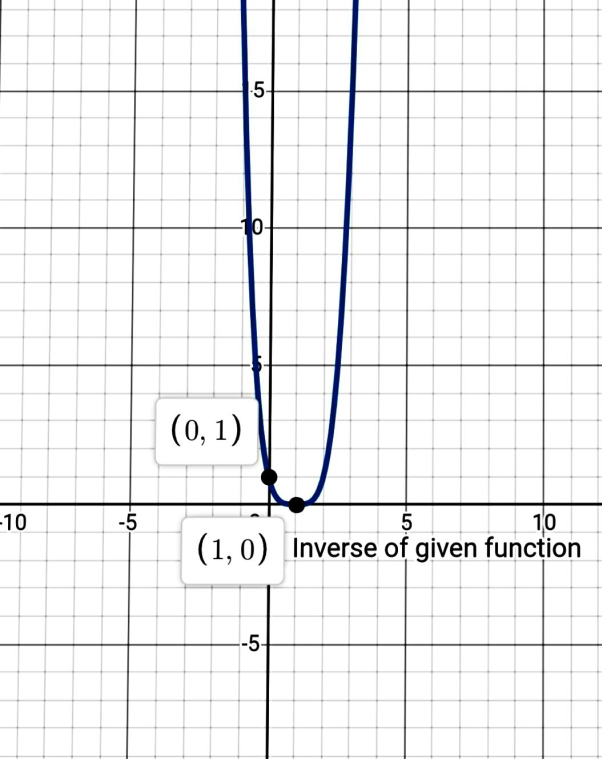# The function f ( x ) = 1 + x 4 is one to one.### Precalculus: Mathematics for Calcu...

6th Edition
Stewart + 5 others
Publisher: Cengage Learning
ISBN: 9780840068071### Precalculus: Mathematics for Calcu...

6th Edition
Stewart + 5 others
Publisher: Cengage Learning
ISBN: 9780840068071

#### Solutions

Chapter 2, Problem 92RE

a.

To determine

## To show: The function f(x)=1+x4 is one to one.

Expert Solution

### Explanation of Solution

Given information:

f(x)=1+x4

To prove a one to one function let us assume that the function is not one to one for f(a) and f(b) where 'a'&'b' are real numbers.

f(a)=1+a4 .....(1)similarly,f(b)=1+b4 .....(2)

Put f(a)=f(b)

1+a4=1+b4a4=b4a=b

This means that value 'a'&'b' is same.

Hence, the function is one to one function.

Hence, proved.

b.

To determine

Expert Solution

### Explanation of Solution

Given information:

f(x)=1+x4

Use graphing device to graph the function f(x)=1+x4.

Graph : Sketch the graph using graphing utility.

Step 1: Press WINDOW button to access the Window editor.

Step 2: Press Y= button.

Step 3: Enter the expression 1+x4 which is required to graph.

Step 4: Press GRAPH button to graph the function and adjust the windows according to the graph.

The graph is obtained as:c.

To determine

Expert Solution

### Explanation of Solution

Given information:

f(x)=1+x4

Use graphing device to graph the function f(x)=1+x4.

Graph : Sketch the graph using graphing utility.

Step 1: Press WINDOW button to access the Window editor.

Step 2: Press Y= button.

Step 3: Enter the expression 1+x4 which is required to graph.

Step 4: Press GRAPH button to graph the function and adjust the windows according to the graph.

The graph is obtained as:d.

To determine

### To find: The inverse of function i.e., f−1(x)

Expert Solution

The inverse of the function is f1(x)=(x1)4

### Explanation of Solution

Given information:

f(x)=1+x4

y=f(x)y=1+x4swap x and yx=1+y4

y4=x1y=(x1)4orf1(x)=(x1)4

Hence, The required equation for the inverse of given function is f1(x)=(x1)4

### Have a homework question?

Subscribe to bartleby learn! Ask subject matter experts 30 homework questions each month. Plus, you’ll have access to millions of step-by-step textbook answers!